## Mensuration - the theory of measurement (plane figures)

Mensuration is the branch of mathematics which deals with the study of various parameters of geometric figures such as areas, perimeters, volumes etc. Engineers often need to know the areas, perimeters and volumes of structures for various reasons.

The most common is that many structures and especially steel structures require some form of surface protection. In order to allow for sufficient quantities of surface protective coatings such as paint and more importantly to know how much a surface coating will cost it is necessary to know the area that needs to be covered.  Other reasons for working out areas etc. might be to order enough gravel for drainage structures such as soakaways or tarmacadam for roads.

The following geometric figures are plane surfaces with only 2 dimensions. Later on, I will include solids and how to work out surface areas and volumes of common figures.

### The Square figure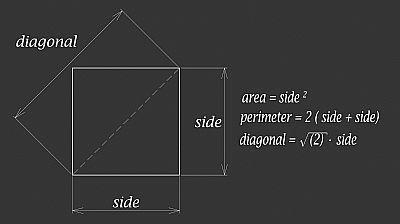Square geometric figure

The geometric figure of the square on the left shows how to calculate the area, perimeter and diagonal sizes.

The area of the square is the two dimensional space that the square covers. To calculate the area of a square the length (l) of two of the sides are multiplied together and the result is given in square units of length.

The perimeter is the total distance around the square. To calculate the perimeter the four sides of the square are added together and the result is given in units of length.

The diagonal length between the top and bottom of the square is calculated using the Pythagorean theorem. This is given as a2 + b2 = c2, by simply replacing c with the diagonal side and a + b with the lengths of the two sides of the square and transposing the equation the length of the diagonal side can be calculated and the result given in units of length.

### The Rectangle figure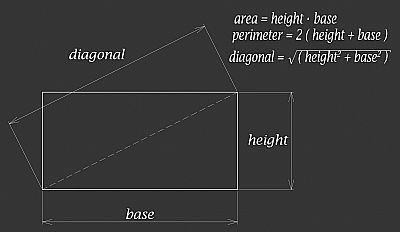Rectangular geometric figure

The geometric figure of the rectangle on the right has the opposite sides parallel with the angles between the base and the height at 90º.

To calculate the area of a rectangle the height and base are multiplied together and the result is given in square units of length.

The total distance around the rectangle is the perimeter and is the distance of the height plus the base doubled. The result is in units of length.

As with the geometric of the square the Pythagorean theorem above is transposed to obtain the square root of the square of the height and square of the base added together.

### The Parallelogram figure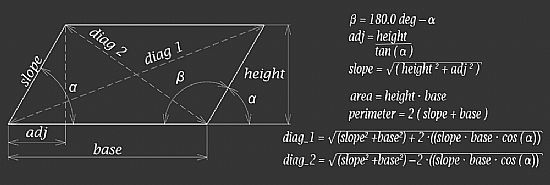Parallelogram geometric figure

The geometric figure of the parallelogram above is a quadrilateral with opposite sides parallel and therefore the opposite angles equal. In order to calculate the various parameters of a parallelogram some preparatory work is necessary. In order to calculate the parameters of a parallelogram the height, base and at least one angle must be known. To find the angle beta if angle alpha is known then the value of alpha needs to be deducted from 180º.

The second preparatory calculation is to work out the adjacent side of the alpha angle. This is the length of the side along the base when a vertical line is drawn from the top angle diametrically opposite the beta angle shown in the diagram. The distance along the base from the alpha angle where the vertical line crosses the base. The adjacent length can be found with trigonometry. The adjacent side is equal to the height of the parallelogram divided by the tangent of angle alpha.

The third preparatory calculation uses the Pythagorean theorem to find the length of the sloping sides of the parallelogram. The area can be calculated the same as for the rectangle ie. height multiplied by the base. The perimeter is estimated by substituting the height in the rectangle calculation above for the sloping side. Finally, the two diagonals are found using almost the same equation, except that a + is replaced by a - in the second equation.

### The Trapezium figure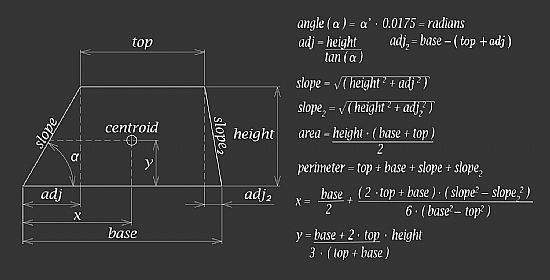Trapezium geometric figure

The geometric figure of the trapezium above, also known as a trapezoid, especially in the USA, is a quadrilateral with top and base parallel. An isosceles trapezium also has two equal angles. In the same way that in order to calculate the various parameters of the parallelogram above some preparatory work is also necessary for obtaining the parameters of the trapezium. In order to calculate the parameters of a trapezium the height, base, top and at least one angle must be known.

The adjacent side of the alpha angle is calculated using trigonometry. The adjacent side of an angle is equal to the opposite side divided by the tangent of the angle. The adjacent side (adj2) of the second base angle is the adj length plus the top length subtracted from the base length of the trapezium. In the case of an isosceles trapezium the adj2 length will be equal to the adj length.

Once these lengths are known both the slope lengths of the trapezium can be calculated using Pythagorean theorem. The geometric parameters of area and perimeter can now be calculated using the equations shown in the diagram above.

Engineers often also need to know the centroid of a structure the centroid is the centre of mass or the geometric centre of the structure. This allows engineers to know where to lift structures so that they are in balance. The simpler geometric figures such as squares, rectangles or parallelograms the centroid is in the centre of the figure, but as the shape becomes more complex, such as a trapezium locating the centroid is more difficult. The x and y co-ordinates of the centroid can be obtained using the equations shown in the diagram above.

### The Triangle figure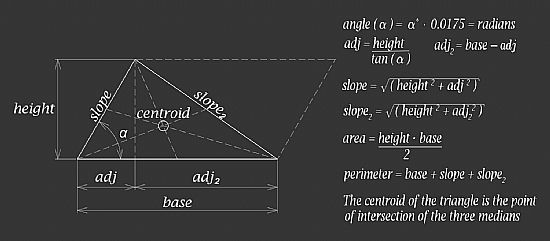Triangle geometric figure

The geometric figure of the triangle to the left is an example of a scalene triangle as it has three sides with different lengths. The other five types of triangles are a right angle triangle that has two acute angles measuring less than 90 degrees and the third angle that is 90 degrees.  An equilateral triangle that has all three sides of equal length and all three angles of equal size. The isosceles triangle has only two of its sides of equal length. An acute triangle has all three of its angles that are less than 90 degrees and finally an obtuse triangle has two angles less than 90 degrees and the third angle is greater than 90 degrees

Like the previous figures if the base, height and one angle of any triangle is known then using trigonometry or Pythagorean theorem it is possible to work out the length of all the sides and angles.

A triangle is half of a parallelogram or in the case of a right angle triangle half a rectangle. The diagram above shows a copy of the triangle has been flipped along the side slope2 shown by the dashed lines. Therefore, the area of any triangle is half the area of a parallelogram or rectangle with the same base and height. From the previous diagrams the area of either a parallelogram or rectangle is the base multiplied by the height. To find the area of a triangle the result of the base multiplied by the height is then divided by two.

The perimeter is worked out by adding the lengths of the base, and two sides together. To find the centroid of a triangle a line can be drawn from the centre of each side to the angle opposite. The point where each of the lines cross will be the centroid of the triangle as shown in the diagram.

### The Circle figure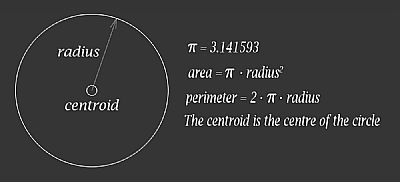Circle geometric figure

The geometric figure on the right is a circle. As long as the radius of the circle is known the area and perimeter or circumference of the circle can be calculated.

The ratio of a circles circumference to its diameter is represented by the 16 letter of the Greek alphabet Pi (π). Pi is a mathematical constant approximately equal to 3.141593.

To calculate the interior area of the circular figure the radius squared is multiplied by Pi. To obtain the circumference or perimeter of the circle the radius is multiplied by Pi and the result doubled.

The centroid of a circular figure is a point equidistant from any point on the perimeter of the circle.

### The Sector of circle figure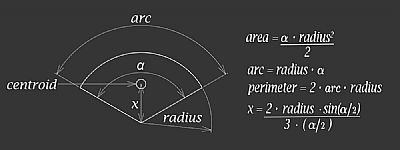Sector of circle geometric figure

The geometric figure on the left is a sector of a circle. The area bounded by the sector is a small portion of the circle between two radii and separated by the arc length on the circle perimeter. The area of the sector is proportional to the arc length. As long as the radius of the circle is known the area can be calculated.  In order to calculate the perimeter, the arc length must be calculated first and to work out the arc length the central angle α must be known.

The centroid is a point equidistant from the radii and is on a line between the centre of the arc length and the central angle at distance x from the central angle.

### The Ellipse figure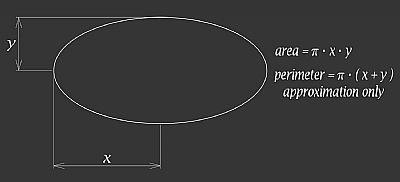Ellipse geometric figure

The geometric figure on the right is an ellipse. The ellipse can be described as the curve around two focal points, such that the sum of the distances of the two focal points is always the same at any point on the ellipse. In order to calculate the area of an ellipse the semi-major axis (x) and the semi-minor axis (y) are required.The perimeter of an ellipse is more difficult to calculate accurately and engineers generally use an approximation. There are lots of different equations to approximate the perimeter of an ellipse. One commonly used is shown in the diagram opposite. I have also created an SMATH calculation document and have included a second approximation for the perimeter in this calculation sheet. The most accurate method is to visualise the ellipse as a continuous curve and therefore integration calculus can be used to determine the length of the curve. I have included the calculus equations in the SMATH document. The centroid of the ellipse is the intersection of the major axis and the minor axis.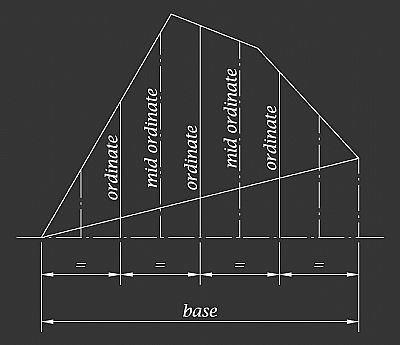Irregular geometric figure

### Irregular plane figure

There are a number of different methods of approximating the area of an irregular plane figure. These include the Mid Ordinate Rule, the Trapezoidal Rule and the Simpson’s Rule. Which ever method is chosen, the starting point is to divide the area into an even number of equal strips. The length of each strip or ordinate is then accurately measured, if either the trapezoidal or Simpson’s rule is used. The length of the mid ordinates is measured if the mid ordinate rule is used. The equal width of each strip is measured and then one of a number of rules can be used to determine the area.

The Mid Ordinate Rule

As its name suggests this rule uses the length of the mid ordinate of each strip to approximate the area.

Area = Width of each ordinate x (sum of all the mid ordinates)

An alternative is to obtain the average of the base length multiplied by the sum of all the mid ordinates.

Area = (length of the base x (sum of all the mid ordinates))/number of strips

The Trapezoidal Rule

Area = width of each strip x ((sum of first and last ordinate)/2) + (sum of the remaining ordinates)

The Simpson’s Rule

Area = (width of each ordinate/3) x ((first + last ordinate) + 4 x (sum of evens) + 2 x (sum of odds).

Intergration

An alternative to the above methods is to use integration to determine the area of an irregular figure. This is particularly useful if the irregular figure contains curves within the border.

The perimeter of an irregular figure can be calculated by breaking the shape down into regular figures such rectangles, squares and sectors of a circle. The perimeter can then be worked out by obtaining the perimeter of the sides that are related to the border of the irregular figure and then adding these together to determine the perimeter of the irregular figure.

### The SMath calculation sheet

I have produced SMath calculation sheets to provide an active model to allow variables to be changed to see how the area, perimeter and centroid of the figures above are calculated. The SMath calculation sheet for the regular figures above can be found on my SMath Cloud web space by clicking on the left hand images below. The irregular figure above can be found by clicking on the right hand images below :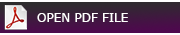View PDF file of regular figures calculation sheet View PDF file of irregular figure calculation sheet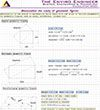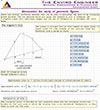Open SMath regular figures calculation sheet Open SMath irregular figure calculation sheet

### Sources used and further reading

Wikipedia Mensuration

Oxford dictonary - Mensuration

Wikipedia - Trapezium

Wikipedia - Ellipse

Mathworld Wolfram - Ellipse